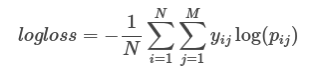# scikit-learn: First steps with log_loss

Over the last week I've spent a little bit of time playing around with the data in the Kaggle TalkingData Mobile User Demographics competition, and came across a notebook written by dune_dweller showing how to run a logistic regression algorithm on the dataset.

The metric used to evaluate the output in this competition is multi class logarithmic loss, which is implemented by the log_loss function in the scikit-learn library.

I've not used it before so I created a small example to get to grips with it.

Let's say we have 3 rows to predict and we happen to know that they should be labelled 'bam', 'spam', and 'ham' respectively:

``````
>>> actual_labels = ["bam", "ham", "spam"]
``````

To work out the log loss score we need to make a prediction for what we think each label actually is. We do this by passing an array containing a probability between 0-1 for each label

e.g. if we think the first label is definitely 'bam' then we'd pass [1, 0, 0], whereas if we thought it had a 50-50 chance of being 'bam' or 'spam' then we might pass [0.5, 0, 0.5]. As far as I can tell the values get sorted into (alphabetical) order so we need to provide our predictions in the same order.

Let's give it a try. First we'll import the function:

``````
>>> from sklearn.metrics import log_loss
``````

Now let's see what score we get if we make a perfect prediction:

``````
>>> log_loss(actual_labels,  [[1, 0, 0], [0, 1, 0], [0, 0, 1]])
2.1094237467877998e-15
``````

What about if we make a completely wrong prediction?

``````
>>> log_loss(actual_labels,  [[0, 0, 1], [1, 0, 0], [0, 1, 0]])
34.538776394910684
``````

We can reverse engineer this score to work out the probability that we've predicted the correct class.

If we look at the case where the average log loss exceeds 1, it is when log(pij) < -1 when i is the true class.

This means that the predicted probability for that given class would be less than exp(-1) or around 0.368.

So, seeing a log loss greater than one can be expected in the cass that that your model only gives less than a 36% probability estimate for the correct class.

This is the formula of logloss:In which yij is 1 for the correct class and 0 for other classes and pij is the probability assigned for that class.

The interesting thing about this formula is that we only care about the correct class. The yij value of 0 cancels out the wrong classes.

In our two examples so far we actually already know the probability estimate for the correct class - 100% in the first case and 0% in the second case, but we can plug in the numbers to check we end up with the same result.

First we need to work out what value would have been passed to the log function which is easy in this case. The value of yij is

``````
# every prediction exactly right
>>> math.log(1)
0.0

>>> math.exp(0)
1.0
``````
``````
# every prediction completely wrong
>>> math.log(0.000000001)
-20.72326583694641

>>> math.exp(-20.72326583694641)
1.0000000000000007e-09
``````

I used a really small value instead of 0 in the second example because math.log(0) trends towards negative infinity.

Let's try another example where we have less certainty:

``````
>>> print log_loss(actual_labels, [[0.8, 0.1, 0.1], [0.3, 0.6, 0.1], [0.15, 0.15, 0.7]])
0.363548039673
``````

We'll have to do a bit more work to figure out what value was being passed to the log function this time, but not too much. This is roughly the calculation being performed:

``````
# 0.363548039673 = -1/3 * (log(0.8) + log(0.6) + log(0.7)

>>> print log_loss(actual_labels,  [[0.8, 0.1, 0.1], [0.3, 0.6, 0.1], [0.15, 0.15, 0.7]])
0.363548039673
``````

In this case, on average our probability estimate would be:

``````
# we put in the negative value since we multiplied by -1/N
>>> math.exp(-0.363548039673)
0.6952053289772744
``````

We had 60%, 70%, and 80% accuracy for our 3 labels so an overall probability of 69.5% seems about right.

One more example. This time we'll make one more very certain (90%) prediction for 'spam':

``````
>>> print log_loss(["bam", "ham", "spam", "spam"], [[0.8, 0.1, 0.1], [0.3, 0.6, 0.1], [0.15, 0.15, 0.7], [0.05, 0.05, 0.9]])
0.299001158669

>>> math.exp(-0.299001158669)
0.741558550213609
``````

74% accuracy overall, sounds about right!